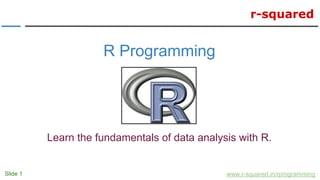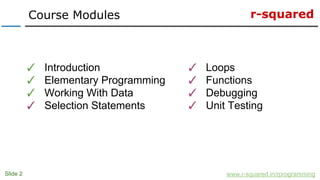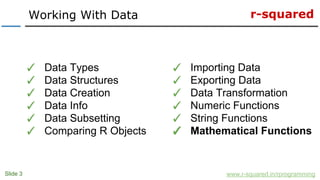Se está descargando tu SlideShare. ×

# R Programming: Mathematical Functions In R

Anuncio
Anuncio
Anuncio
Anuncio
Anuncio
Anuncio
Anuncio
Anuncio
Anuncio
Anuncio
AnuncioCargando en…3
×

1 de 24 Anuncio

# R Programming: Mathematical Functions In R

Learn the built-in mathematical functions in R. This tutorial is part of the Working With Data module of the R Programming course offered by r-squared.

Learn the built-in mathematical functions in R. This tutorial is part of the Working With Data module of the R Programming course offered by r-squared.

Anuncio
Anuncio

### R Programming: Mathematical Functions In R

1. 1. r-squared Slide 1 www.r-squared.in/rprogramming R Programming Learn the fundamentals of data analysis with R.
2. 2. r-squared Slide 2 Course Modules www.r-squared.in/rprogramming ✓ Introduction ✓ Elementary Programming ✓ Working With Data ✓ Selection Statements ✓ Loops ✓ Functions ✓ Debugging ✓ Unit Testing
3. 3. r-squared Slide 3 Working With Data www.r-squared.in/rprogramming ✓ Data Types ✓ Data Structures ✓ Data Creation ✓ Data Info ✓ Data Subsetting ✓ Comparing R Objects ✓ Importing Data ✓ Exporting Data ✓ Data Transformation ✓ Numeric Functions ✓ String Functions ✓ Mathematical Functions
4. 4. r-squared In this unit, we will explore the following mathematical functions: Slide 4 Mathematical Functions www.r-squared.in/rprogramming ● Arithmetic Operators ● Column/ Row Operators ● Cumulative Operators ● Sampling ● Set Operations ● Logarithm
5. 5. r-squared Slide 5 Arithmetic Operators www.r-squared.in/rprogramming Operator Description + Addition - Subtraction * Multiplication / Division ^ Exponential %% Modulus %/% Integer division
6. 6. r-squared Slide 6 Arithmetic Operators www.r-squared.in/rprogramming Examples > # example 1 > x <- 5 > y <- 2 > x + y  7 > x - y  3 > x * y  10 > x / y  2.5
7. 7. r-squared Slide 7 Arithmetic Operators www.r-squared.in/rprogramming Examples > # example 2 > x <- 5 > y <- 2 > x ^ y  25 > x %% y  1 > x %/% y  2
8. 8. r-squared Slide 8 Column & Row Operations www.r-squared.in/rprogramming Operator Description colSums Sum of column values rowSums Sum of row values colMeans Mean of column values rowMeans Mean of row values
9. 9. r-squared Slide 9 Column & Row Operations www.r-squared.in/rprogramming Examples > # example 1 > m [,1] [,2] [,3] [1,] 1 4 7 [2,] 2 5 8 [3,] 3 6 9 > colSums(m) # sum of columns  6 15 24 > rowSums(m) # sum of rows  12 15 18 > colMeans(m) # mean of columns  2 5 8 > rowMeans(m) # mean of rows  4 5 6
10. 10. r-squared Slide 10 Cumulative Operations www.r-squared.in/rprogramming Operator Description cumsum Cumulative sums cumprod Cumulative products cummin Cumulative minima cummax Cumulative maxima
11. 11. r-squared Slide 11 Cumulative Operations www.r-squared.in/rprogramming Examples > # example 1 > x <- 1:5 > cumsum(x)  1 3 6 10 15 > cumprod(x)  1 2 6 24 120 > x <- c(3:1, 2:0, 4:2) > cummin(x)  3 2 1 1 1 0 0 0 0 > cummax(x)  3 3 3 3 3 3 4 4 4
12. 12. r-squared Slide 12 Miscellaneous Functions www.r-squared.in/rprogramming Operator Description max Maxima min Minima pmax Parallel minima pmin Parallel maxima sum Sum of the values prod Product of the values range Min and Max of the values
13. 13. r-squared Slide 13 Miscellaneous Functions www.r-squared.in/rprogramming Examples > # example 1 > x <- c(3, 26, 122, 6) > min(x)  3 > max(x)  122 > prod(x)  57096 > sum(x)  157 > range(x)  3 122
14. 14. r-squared Slide 14 Miscellaneous Functions www.r-squared.in/rprogramming Examples > # example 2 > x <- c(3, 26, 122, 6) > y <- c(43,2,54,8) > z <- c(9,32,1,9) > pmin(x, y, z)  3 2 1 6 > pmax(x, y, z)  43 32 122 9
15. 15. r-squared Slide 15 sample() www.r-squared.in/rprogramming Description sample() takes a sample of the specified size from the elements of an object, with or without replacement. Syntax sample(x, size, replace = FALSE, prob = NULL) Returns Sample of the specified size. Documentation help(sample)
16. 16. r-squared Slide 16 sample() www.r-squared.in/rprogramming Examples > example 1 > x <- 1:10 > sample(x)  10 1 4 2 9 7 5 6 8 3 > sample(10)  7 6 10 8 2 9 1 3 4 5 > sample(x, size = 5)  1 10 8 9 5
17. 17. r-squared Slide 17 sample() www.r-squared.in/rprogramming Examples > example 2 > c <- c("Heads", "Tails") > sample(c, size = 1)  "Heads" > sample(c, size = 2)  "Tails" "Heads" > sample(c, size = 10, replace = TRUE)  "Tails" "Tails" "Tails" "Heads" "Heads" "Heads" "Tails" "Heads" "Heads" "Heads" > table(sample(c, size = 10, replace = TRUE)) Heads Tails 5 5
18. 18. r-squared Slide 18 Set Operations www.r-squared.in/rprogramming Perform set operations on two vectors Operation Description union(x, y) Union of x and y intersect(x, y) Intersect of x and y setdiff(x, y) Elements in x and not in y setequal(x, y) Test if x and y are equal
19. 19. r-squared Slide 19 Set Operations www.r-squared.in/rprogramming Examples > example 1 > x <- 1:10 > y <- 6:15 > union(x, y)  1 2 3 4 5 6 7 8 9 10 11 12 13 14 15 > intersect(x, y)  6 7 8 9 10 > setdiff(x, y)  1 2 3 4 5 > setdiff(y, x)  11 12 13 14 15 > setequal(x, y)  FALSE
20. 20. r-squared Slide 20 Logarithm www.r-squared.in/rprogramming Description log() computes the natural logarithm. Syntax log(x, base) Returns Logarithm of the specified base or the natural logarithm Documentation help(log) help(exp)
21. 21. r-squared Slide 21 log() www.r-squared.in/rprogramming Examples > example 1 > log(10, base = 10)  1 > log(10, base = 2)  3.321928 > log(2.71828)  0.9999993 > # natural logarithm > log(10)  2.302585 > # base 10 > log10(10)  1
22. 22. r-squared Slide 22 log() www.r-squared.in/rprogramming Examples > # base 2 > log2(10)  3.321928 > # exponential > exp(3)  20.08554 > 2.71828 ^ 3  20.0855
23. 23. r-squared In the next module, we will explore selection statements in R: Slide 23 Next Steps... www.r-squared.in/rprogramming ● if() ● if else() ● ifelse() ● switch()
24. 24. r-squared Slide 24 Connect With Us www.r-squared.in/rprogramming Visit r-squared for tutorials on: ● R Programming ● Business Analytics ● Data Visualization ● Web Applications ● Package Development ● Git & GitHub